# A fast implementation of matrix-matrix product in double-double precision on NVIDIA C2050 and application to semidefinite programming

Dec. 6, 2012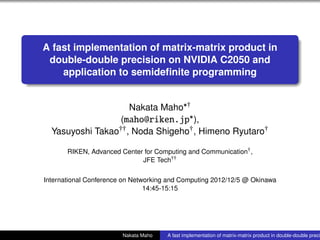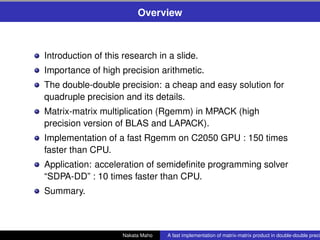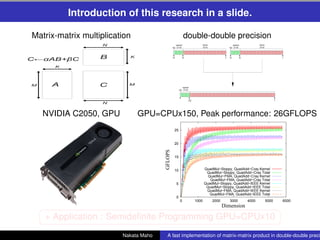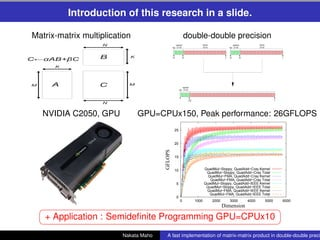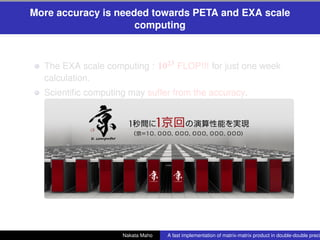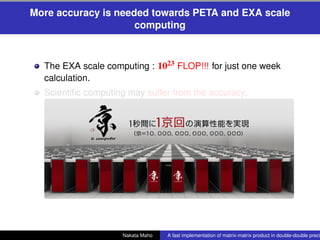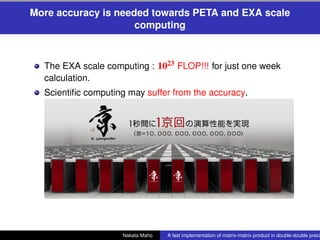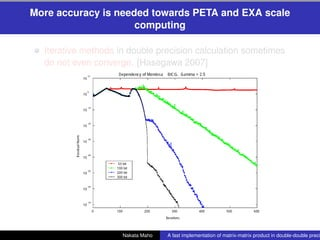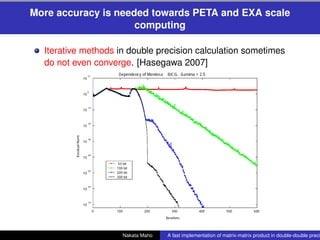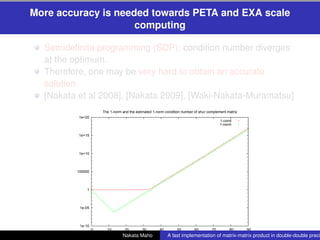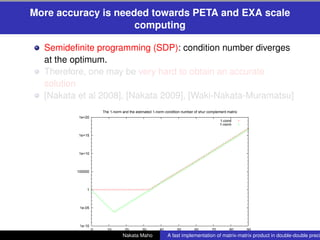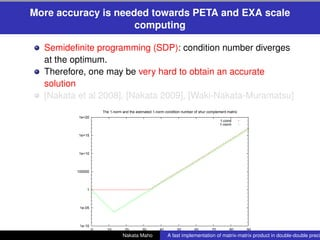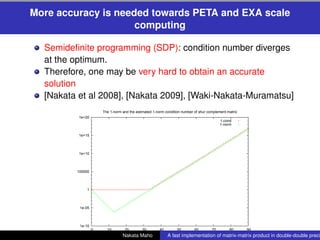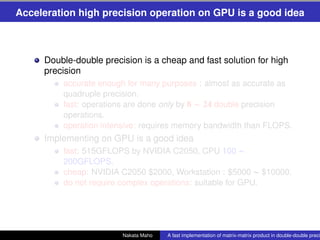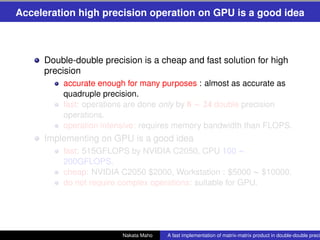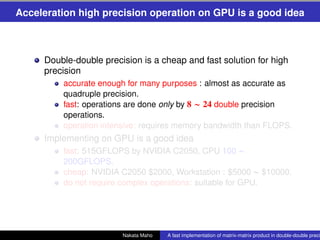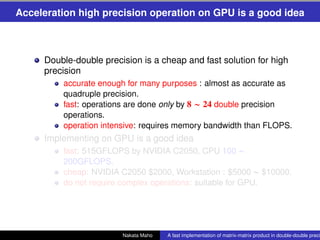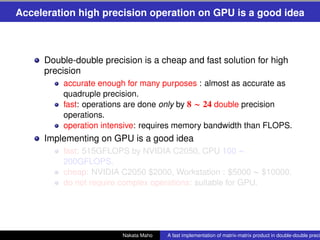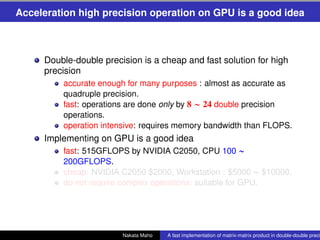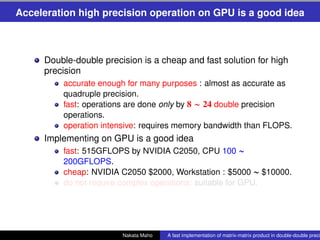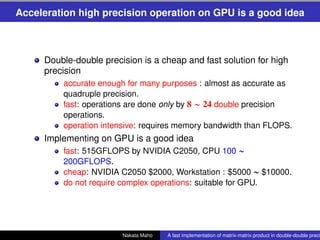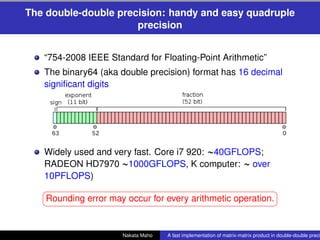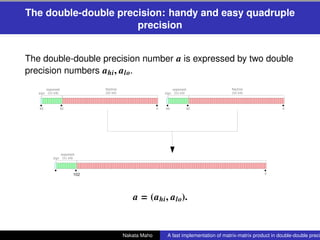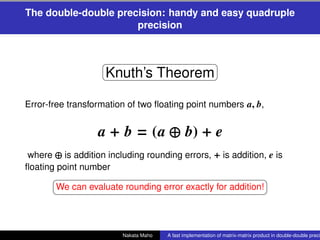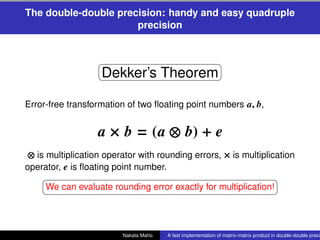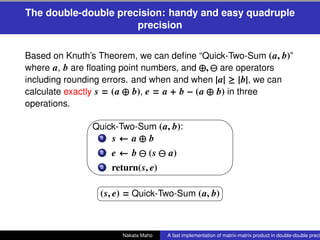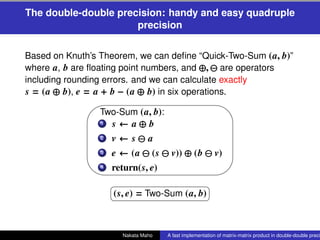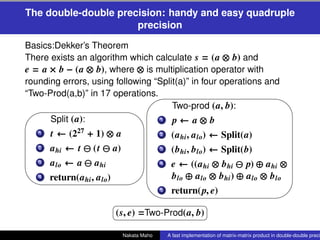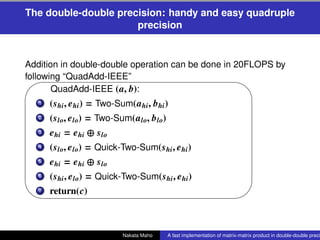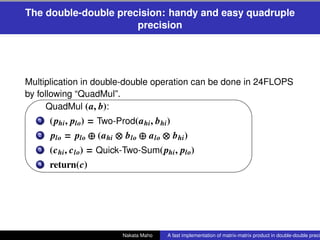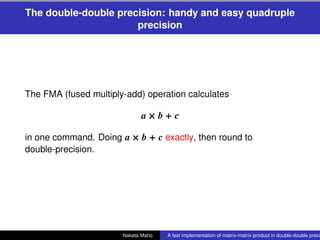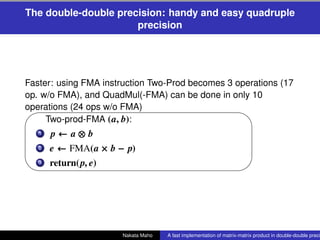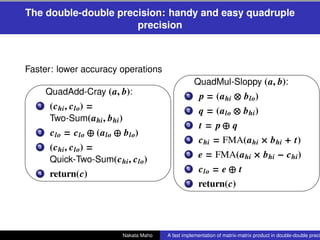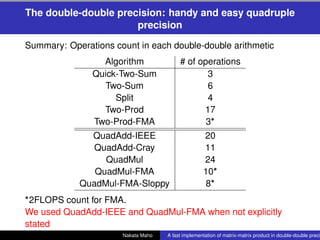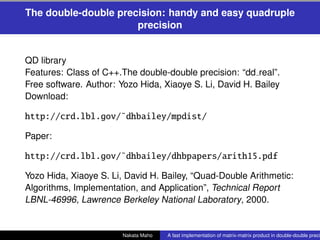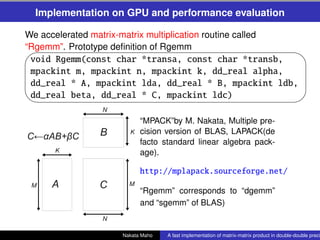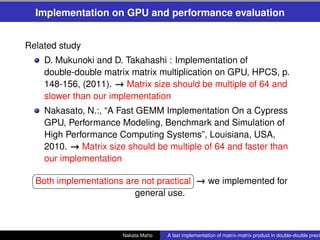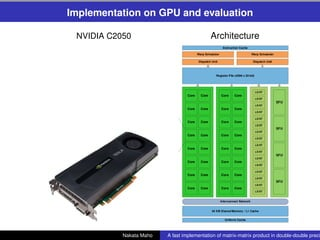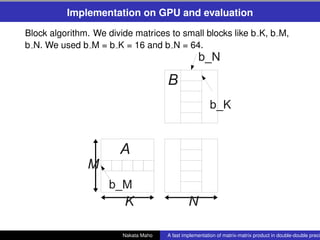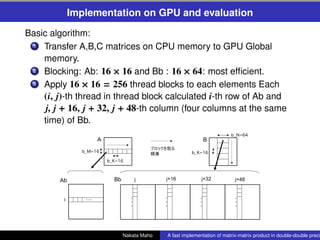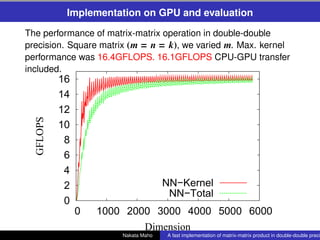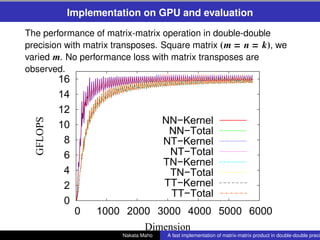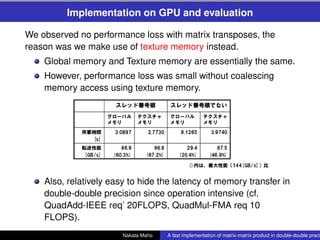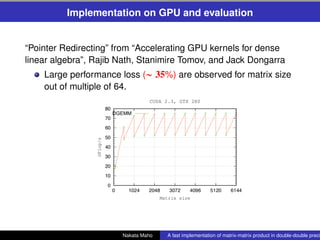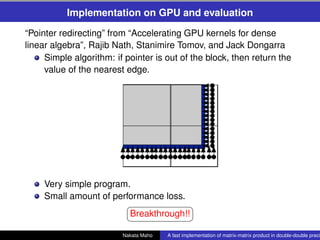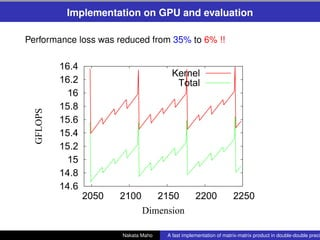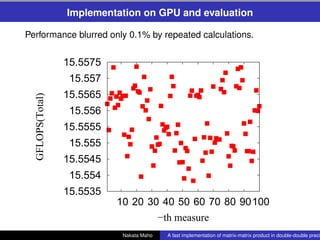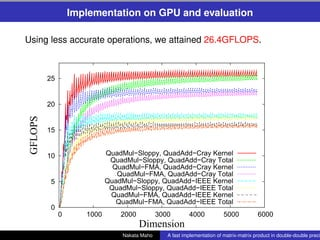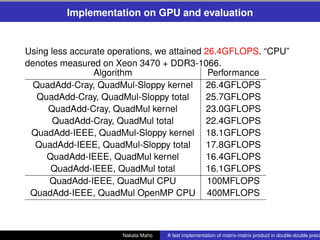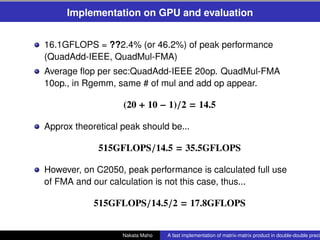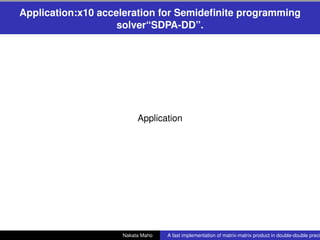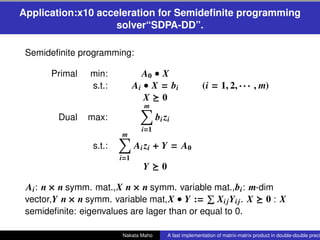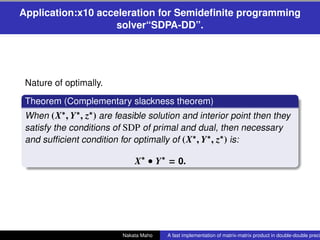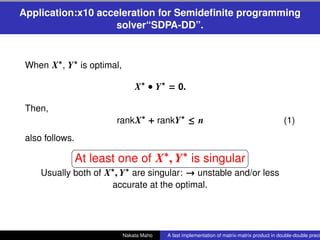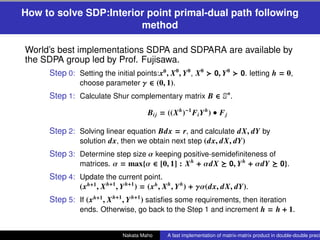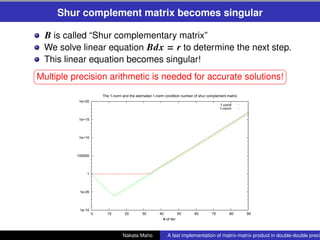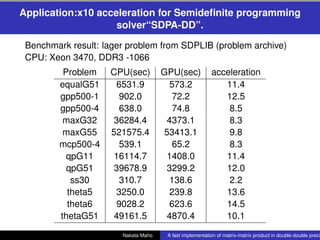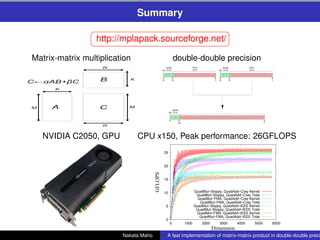1 of 59

### A fast implementation of matrix-matrix product in double-double precision on NVIDIA C2050 and application to semidefinite programming

• 1. . A fast implementation of matrix-matrix product in double-double precision on NVIDIA C2050 and application to semideﬁnite programming . Nakata Maho∗† (maho@riken.jp∗ ), Yasuyoshi Takao†† , Noda Shigeho† , Himeno Ryutaro† RIKEN, Advanced Center for Computing and Communication† , JFE Tech†† International Conference on Networking and Computing 2012/12/5 @ Okinawa 14:45-15:15 Nakata Maho A fast implementation of matrix-matrix product in double-double preci
• 2. Overview Introduction of this research in a slide. Importance of high precision arithmetic. The double-double precision: a cheap and easy solution for quadruple precision and its details. Matrix-matrix multiplication (Rgemm) in MPACK (high precision version of BLAS and LAPACK). Implementation of a fast Rgemm on C2050 GPU : 150 times faster than CPU. Application: acceleration of semideﬁnite programming solver “SDPA-DD” : 10 times faster than CPU. Summary. Nakata Maho A fast implementation of matrix-matrix product in double-double preci
• 5. More accuracy is needed towards PETA and EXA scale computing The EXA scale computing : 1023 FLOP!!! for just one week calculation. Scientiﬁc computing may suffer from the accuracy. Nakata Maho A fast implementation of matrix-matrix product in double-double preci
• 6. More accuracy is needed towards PETA and EXA scale computing The EXA scale computing : 1023 FLOP!!! for just one week calculation. Scientiﬁc computing may suffer from the accuracy. Nakata Maho A fast implementation of matrix-matrix product in double-double preci
• 7. More accuracy is needed towards PETA and EXA scale computing The EXA scale computing : 1023 FLOP!!! for just one week calculation. Scientiﬁc computing may suffer from the accuracy. Nakata Maho A fast implementation of matrix-matrix product in double-double preci
• 8. More accuracy is needed towards PETA and EXA scale computing Iterative methods in double precision calculation sometimes do not even converge. [Hasegawa 2007] Nakata Maho A fast implementation of matrix-matrix product in double-double preci
• 9. More accuracy is needed towards PETA and EXA scale computing Iterative methods in double precision calculation sometimes do not even converge. [Hasegawa 2007] Nakata Maho A fast implementation of matrix-matrix product in double-double preci
• 10. More accuracy is needed towards PETA and EXA scale computing Semideﬁnite programming (SDP): condition number diverges at the optimum. Therefore, one may be very hard to obtain an accurate solution [Nakata et al 2008], [Nakata 2009], [Waki-Nakata-Muramatsu] The 1-norm and the estimated 1-norm condition number of shur complement matrix 1e+20 1-cond 1-norm 1e+15 1e+10 100000 1 1e-05 1e-10 0 10 20 30 40 50 60 70 80 90 Nakata Maho # of iter. implementation of A fast matrix-matrix product in double-double preci
• 11. More accuracy is needed towards PETA and EXA scale computing Semideﬁnite programming (SDP): condition number diverges at the optimum. Therefore, one may be very hard to obtain an accurate solution [Nakata et al 2008], [Nakata 2009], [Waki-Nakata-Muramatsu] The 1-norm and the estimated 1-norm condition number of shur complement matrix 1e+20 1-cond 1-norm 1e+15 1e+10 100000 1 1e-05 1e-10 0 10 20 30 40 50 60 70 80 90 Nakata Maho # of iter. implementation of A fast matrix-matrix product in double-double preci
• 12. More accuracy is needed towards PETA and EXA scale computing Semideﬁnite programming (SDP): condition number diverges at the optimum. Therefore, one may be very hard to obtain an accurate solution [Nakata et al 2008], [Nakata 2009], [Waki-Nakata-Muramatsu] The 1-norm and the estimated 1-norm condition number of shur complement matrix 1e+20 1-cond 1-norm 1e+15 1e+10 100000 1 1e-05 1e-10 0 10 20 30 40 50 60 70 80 90 Nakata Maho # of iter. implementation of A fast matrix-matrix product in double-double preci
• 13. More accuracy is needed towards PETA and EXA scale computing Semideﬁnite programming (SDP): condition number diverges at the optimum. Therefore, one may be very hard to obtain an accurate solution [Nakata et al 2008], [Nakata 2009], [Waki-Nakata-Muramatsu] The 1-norm and the estimated 1-norm condition number of shur complement matrix 1e+20 1-cond 1-norm 1e+15 1e+10 100000 1 1e-05 1e-10 0 10 20 30 40 50 60 70 80 90 Nakata Maho # of iter. implementation of A fast matrix-matrix product in double-double preci
• 14. Acceleration high precision operation on GPU is a good idea Double-double precision is a cheap and fast solution for high precision accurate enough for many purposes : almost as accurate as quadruple precision. fast: operations are done only by 8 ∼ 24 double precision operations. operation intensive: requires memory bandwidth than FLOPS. Implementing on GPU is a good idea fast: 515GFLOPS by NVIDIA C2050, CPU 100 ∼ 200GFLOPS. cheap: NVIDIA C2050 \$2000, Workstation : \$5000 ∼ \$10000. do not require complex operations: suitable for GPU. Nakata Maho A fast implementation of matrix-matrix product in double-double preci
• 15. Acceleration high precision operation on GPU is a good idea Double-double precision is a cheap and fast solution for high precision accurate enough for many purposes : almost as accurate as quadruple precision. fast: operations are done only by 8 ∼ 24 double precision operations. operation intensive: requires memory bandwidth than FLOPS. Implementing on GPU is a good idea fast: 515GFLOPS by NVIDIA C2050, CPU 100 ∼ 200GFLOPS. cheap: NVIDIA C2050 \$2000, Workstation : \$5000 ∼ \$10000. do not require complex operations: suitable for GPU. Nakata Maho A fast implementation of matrix-matrix product in double-double preci
• 16. Acceleration high precision operation on GPU is a good idea Double-double precision is a cheap and fast solution for high precision accurate enough for many purposes : almost as accurate as quadruple precision. fast: operations are done only by 8 ∼ 24 double precision operations. operation intensive: requires memory bandwidth than FLOPS. Implementing on GPU is a good idea fast: 515GFLOPS by NVIDIA C2050, CPU 100 ∼ 200GFLOPS. cheap: NVIDIA C2050 \$2000, Workstation : \$5000 ∼ \$10000. do not require complex operations: suitable for GPU. Nakata Maho A fast implementation of matrix-matrix product in double-double preci
• 17. Acceleration high precision operation on GPU is a good idea Double-double precision is a cheap and fast solution for high precision accurate enough for many purposes : almost as accurate as quadruple precision. fast: operations are done only by 8 ∼ 24 double precision operations. operation intensive: requires memory bandwidth than FLOPS. Implementing on GPU is a good idea fast: 515GFLOPS by NVIDIA C2050, CPU 100 ∼ 200GFLOPS. cheap: NVIDIA C2050 \$2000, Workstation : \$5000 ∼ \$10000. do not require complex operations: suitable for GPU. Nakata Maho A fast implementation of matrix-matrix product in double-double preci
• 18. Acceleration high precision operation on GPU is a good idea Double-double precision is a cheap and fast solution for high precision accurate enough for many purposes : almost as accurate as quadruple precision. fast: operations are done only by 8 ∼ 24 double precision operations. operation intensive: requires memory bandwidth than FLOPS. Implementing on GPU is a good idea fast: 515GFLOPS by NVIDIA C2050, CPU 100 ∼ 200GFLOPS. cheap: NVIDIA C2050 \$2000, Workstation : \$5000 ∼ \$10000. do not require complex operations: suitable for GPU. Nakata Maho A fast implementation of matrix-matrix product in double-double preci
• 19. Acceleration high precision operation on GPU is a good idea Double-double precision is a cheap and fast solution for high precision accurate enough for many purposes : almost as accurate as quadruple precision. fast: operations are done only by 8 ∼ 24 double precision operations. operation intensive: requires memory bandwidth than FLOPS. Implementing on GPU is a good idea fast: 515GFLOPS by NVIDIA C2050, CPU 100 ∼ 200GFLOPS. cheap: NVIDIA C2050 \$2000, Workstation : \$5000 ∼ \$10000. do not require complex operations: suitable for GPU. Nakata Maho A fast implementation of matrix-matrix product in double-double preci
• 20. Acceleration high precision operation on GPU is a good idea Double-double precision is a cheap and fast solution for high precision accurate enough for many purposes : almost as accurate as quadruple precision. fast: operations are done only by 8 ∼ 24 double precision operations. operation intensive: requires memory bandwidth than FLOPS. Implementing on GPU is a good idea fast: 515GFLOPS by NVIDIA C2050, CPU 100 ∼ 200GFLOPS. cheap: NVIDIA C2050 \$2000, Workstation : \$5000 ∼ \$10000. do not require complex operations: suitable for GPU. Nakata Maho A fast implementation of matrix-matrix product in double-double preci
• 21. Acceleration high precision operation on GPU is a good idea Double-double precision is a cheap and fast solution for high precision accurate enough for many purposes : almost as accurate as quadruple precision. fast: operations are done only by 8 ∼ 24 double precision operations. operation intensive: requires memory bandwidth than FLOPS. Implementing on GPU is a good idea fast: 515GFLOPS by NVIDIA C2050, CPU 100 ∼ 200GFLOPS. cheap: NVIDIA C2050 \$2000, Workstation : \$5000 ∼ \$10000. do not require complex operations: suitable for GPU. Nakata Maho A fast implementation of matrix-matrix product in double-double preci
• 22. The double-double precision: handy and easy quadruple precision “754-2008 IEEE Standard for Floating-Point Arithmetic” The binary64 (aka double precision) format has 16 decimal signiﬁcant digits Widely used and very fast. Core i7 920: ∼40GFLOPS; RADEON HD7970 ∼1000GFLOPS, K computer: ∼ over 10PFLOPS) § ¤ Rounding error may occur for every arithmetic operation. ¦ ¥ Nakata Maho A fast implementation of matrix-matrix product in double-double preci
• 23. The double-double precision: handy and easy quadruple precision The double-double precision number a is expressed by two double precision numbers a hi , a lo. a = (a hi , a lo). Nakata Maho A fast implementation of matrix-matrix product in double-double preci
• 24. The double-double precision: handy and easy quadruple precision § ¤ Knuth’s Theorem ¥ ¦ Error-free transformation of two ﬂoating point numbers a, b, a + b = (a ⊕ b) + e where ⊕ is addition including rounding errors, + is addition, e is ﬂoating point number § ¤ We can evaluate rounding error exactly for addition! ¦ ¥ Nakata Maho A fast implementation of matrix-matrix product in double-double preci
• 25. The double-double precision: handy and easy quadruple precision § ¤ Dekker’s Theorem ¥ ¦ Error-free transformation of two ﬂoating point numbers a, b, a × b = (a ⊗ b) + e ⊗ is multiplication operator with rounding errors, × is multiplication operator, e is ﬂoating point number. § ¤ We can evaluate rounding error exactly for multiplication! ¦ ¥ Nakata Maho A fast implementation of matrix-matrix product in double-double preci
• 26. The double-double precision: handy and easy quadruple precision Based on Knuth’s Theorem, we can deﬁne “Quick-Two-Sum (a, b)” where a, b are ﬂoating point numbers, and ⊕, are operators including rounding errors. and when and when |a| ≥ |b|, we can calculate exactly s = (a ⊕ b), e = a + b − (a ⊕ b) in three operations. 1 ( Quick-Two-Sum (a, b): 1. s← a⊕b . e ← b (s a) 2 3. return(s, e) 0 ) § ¤ (s, e) = Quick-Two-Sum (a, b) ¥ ¦ Nakata Maho A fast implementation of matrix-matrix product in double-double preci
• 27. The double-double precision: handy and easy quadruple precision Based on Knuth’s Theorem, we can deﬁne “Quick-Two-Sum (a, b)” where a, b are ﬂoating point numbers, and ⊕, are operators including rounding errors. and we can calculate exactly s = (a ⊕ b), e = a + b − (a ⊕ b) in six operations. 9 6 Two-Sum (a, b): 1. s← a⊕b . v←s a 2 3. e ← (a (s v)) ⊕ (b v) 4. return(s, e) 8 7 § ¤ (s, e) = Two-Sum (a, b) ¥ ¦ Nakata Maho A fast implementation of matrix-matrix product in double-double preci
• 28. The double-double precision: handy and easy quadruple precision Basics:Dekker’s Theorem There exists an algorithm which calculate s = (a ⊗ b) and e = a × b − (a ⊗ b), where ⊗ is multiplication operator with rounding errors, using following “Split(a)” in four operations and “Two-Prod(a,b)” in 17 operations. 9 6 9 6 Two-prod (a, b): Split (a): . p← a⊗b 1 1. t ← (227 + 1) ⊗ a . (a , a ) ← Split(a) 2 hi lo . a hi ← t (t a) 2 . (b hi , b lo) ← Split(b) 3 3. a lo ← a a hi . e ← ((a hi ⊗ b hi p) ⊕ a hi ⊗ 4 4. return(a hi , a lo) b lo ⊕ a lo ⊗ b hi ) ⊕ a lo ⊗ b lo 8 7 . return( p, e) 5 8 7 § ¤ (s, e) =Two-Prod(a, b) ¥ ¦ Nakata Maho A fast implementation of matrix-matrix product in double-double preci
• 29. The double-double precision: handy and easy quadruple precision Addition in double-double operation can be done in 20FLOPS by following “QuadAdd-IEEE” 9 6 QuadAdd-IEEE (a, b): 1. (s hi , e hi ) = Two-Sum(a hi , b hi ) 2. (s lo, e lo) = Two-Sum(a lo, b lo) 3. e hi = e hi ⊕ s lo 4. (s lo, e lo) = Quick-Two-Sum(s hi , e hi ) 5. e hi = e hi ⊕ s lo . (s hi , e lo) = Quick-Two-Sum(s hi , e hi ) 6 7. return(c) 8 7 Nakata Maho A fast implementation of matrix-matrix product in double-double preci
• 30. The double-double precision: handy and easy quadruple precision Multiplication in double-double operation can be done in 24FLOPS by following “QuadMul”. 9 6 QuadMul (a, b): 1. ( phi , plo) = Two-Prod(a hi , b hi ) 2. plo = plo ⊕ (a hi ⊗ b lo ⊕ a lo ⊗ b hi ) 3. (c hi , c lo) = Quick-Two-Sum(phi , plo) 4. return(c) 8 7 Nakata Maho A fast implementation of matrix-matrix product in double-double preci
• 31. The double-double precision: handy and easy quadruple precision The FMA (fused multiply-add) operation calculates a×b+c in one command. Doing a × b + c exactly, then round to double-precision. Nakata Maho A fast implementation of matrix-matrix product in double-double preci
• 32. The double-double precision: handy and easy quadruple precision Faster: using FMA instruction Two-Prod becomes 3 operations (17 op. w/o FMA), and QuadMul(-FMA) can be done in only 10 operations (24 ops w/o FMA) 1 ( Two-prod-FMA (a, b): 1. p← a⊗b . e ← FMA(a × b − p) 2 3. return(p, e) 0 ) Nakata Maho A fast implementation of matrix-matrix product in double-double preci
• 33. The double-double precision: handy and easy quadruple precision Faster: lower accuracy operations 9 6 9 6 QuadMul-Sloppy (a, b): QuadAdd-Cray (a, b): 1. p = (a hi ⊗ b lo) 1. (c hi , c lo) = 2. q = (a lo ⊗ b hi ) Two-Sum(a hi , b hi ) . t = p⊕ q 3 2. c lo = c lo ⊕ (a lo ⊕ b lo) 4. c hi = FMA(a hi × b hi + t) 3. (c hi , c lo) = 5. e = FMA(a hi × b hi − c hi ) Quick-Two-Sum(c hi , c lo) 6. c lo = e ⊕ t 4. return(c) 8 7 7. return(c) 8 7 Nakata Maho A fast implementation of matrix-matrix product in double-double preci
• 35. The double-double precision: handy and easy quadruple precision QD library Features: Class of C++.The double-double precision: “dd real”. Free software. Author: Yozo Hida, Xiaoye S. Li, David H. Bailey Download: http://crd.lbl.gov/˜dhbailey/mpdist/ Paper: http://crd.lbl.gov/˜dhbailey/dhbpapers/arith15.pdf Yozo Hida, Xiaoye S. Li, David H. Bailey, “Quad-Double Arithmetic: Algorithms, Implementation, and Application”, Technical Report LBNL-46996, Lawrence Berkeley National Laboratory, 2000. Nakata Maho A fast implementation of matrix-matrix product in double-double preci
• 36. Implementation on GPU and performance evaluation We accelerated matrix-matrix multiplication routine called “Rgemm”. Prototype deﬁnition of Rgemm ' \$ void Rgemm(const char *transa, const char *transb, mpackint m, mpackint n, mpackint k, dd_real alpha, dd_real * A, mpackint lda, dd_real * B, mpackint ldb, dd_real beta, dd_real * C, mpackint ldc) & % “MPACK”by M. Nakata, Multiple pre- cision version of BLAS, LAPACK(de facto standard linear algebra pack- age). http://mplapack.sourceforge.net/ “Rgemm” corresponds to “dgemm” and “sgemm” of BLAS) Nakata Maho A fast implementation of matrix-matrix product in double-double preci
• 37. Implementation on GPU and performance evaluation Related study D. Mukunoki and D. Takahashi : Implementation of double-double matrix matrix multiplication on GPU, HPCS, p. 148-156, (2011). → Matrix size should be multiple of 64 and slower than our implementation Nakasato, N.:, “A Fast GEMM Implementation On a Cypress GPU, Performance Modeling, Benchmark and Simulation of High Performance Computing Systems”, Louisiana, USA, 2010. → Matrix size should be multiple of 64 and faster than our implementation § ¤ Both implementations are not practical → we implemented for ¦ ¥ general use. Nakata Maho A fast implementation of matrix-matrix product in double-double preci
• 38. Implementation on GPU and evaluation NVIDIA C2050 Architecture Nakata Maho A fast implementation of matrix-matrix product in double-double preci
• 39. Implementation on GPU and evaluation Block algorithm. We divide matrices to small blocks like b K, b M, b N. We used b M = b K = 16 and b N = 64. Nakata Maho A fast implementation of matrix-matrix product in double-double preci
• 40. Implementation on GPU and evaluation Basic algorithm: 1. Transfer A,B,C matrices on CPU memory to GPU Global memory. 2. Blocking: Ab: 16 × 16 and Bb : 16 × 64: most efﬁcient. 3. Apply 16 × 16 = 256 thread blocks to each elements Each (i, j)-th thread in thread block calculated i-th row of Ab and j, j + 16, j + 32, j + 48-th column (four columns at the same time) of Bb. Nakata Maho A fast implementation of matrix-matrix product in double-double preci
• 41. Implementation on GPU and evaluation Operation of each thread in detail: 1. Multiply beta to c0, c1, c2, c3 of C matrix which correspond to i-th column of Ab and j, j + 16, j + 32, j + 48-th row of Bb. 2. Read the ﬁrst block Ab and Bb from global memory to shared memory. Each thread of blocks read its elements. 3. Calculate inner product of row vector ai of Ab and column bi of Bb bi , bi+16 , bi+32 , bi+48 as p0 , p1 , p2 , p3 4. Update c0, c1, c2, c3 like c0 ← c0 + α p0. 5. Read next blocks Ab, Bb and repeat 3, 4, until no further blocks are available. 6. Update C-matrix by c0, c1, c2, c3. 7. Finally transfer C-matrix from GPU Global memory to CPU. Nakata Maho A fast implementation of matrix-matrix product in double-double preci
• 42. Implementation on GPU and evaluation The performance of matrix-matrix operation in double-double precision. Square matrix (m = n = k), we varied m. Max. kernel performance was 16.4GFLOPS. 16.1GFLOPS CPU-GPU transfer included. 16 14 12 GFLOPS 10 8 6 4 2 NN−Kernel NN−Total 0 0 1000 2000 3000 4000 5000 6000 Dimension Nakata Maho A fast implementation of matrix-matrix product in double-double preci
• 43. Implementation on GPU and evaluation The performance of matrix-matrix operation in double-double precision with matrix transposes. Square matrix (m = n = k), we varied m. No performance loss with matrix transposes are observed. 16 14 12 NN−Kernel GFLOPS 10 NN−Total 8 NT−Kernel 6 NT−Total TN−Kernel 4 TN−Total 2 TT−Kernel TT−Total 0 0 1000 2000 3000 4000 5000 6000 Dimension Nakata Maho A fast implementation of matrix-matrix product in double-double preci
• 44. Implementation on GPU and evaluation We observed no performance loss with matrix transposes, the reason was we make use of texture memory instead. Global memory and Texture memory are essentially the same. However, performance loss was small without coalescing memory access using texture memory. Also, relatively easy to hide the latency of memory transfer in double-double precision since operation intensive (cf. QuadAdd-IEEE req’ 20FLOPS, QuadMul-FMA req 10 FLOPS). Nakata Maho A fast implementation of matrix-matrix product in double-double preci
• 45. Implementation on GPU and evaluation “Pointer Redirecting” from “Accelerating GPU kernels for dense linear algebra”, Rajib Nath, Stanimire Tomov, and Jack Dongarra Large performance loss (∼ 35%) are observed for matrix size out of multiple of 64. Nakata Maho A fast implementation of matrix-matrix product in double-double preci
• 46. Implementation on GPU and evaluation “Pointer redirecting” from “Accelerating GPU kernels for dense linear algebra”, Rajib Nath, Stanimire Tomov, and Jack Dongarra Simple algorithm: if pointer is out of the block, then return the value of the nearest edge. Very simple program. Small amount of performance loss. § ¤ Breakthrough!! ¦ ¥ Nakata Maho A fast implementation of matrix-matrix product in double-double preci
• 47. Implementation on GPU and evaluation Performance loss was reduced from 35% to 6% !! 16.4 Kernel 16.2 Total 16 15.8 GFLOPS 15.6 15.4 15.2 15 14.8 14.6 2050 2100 2150 2200 2250 Dimension Nakata Maho A fast implementation of matrix-matrix product in double-double preci
• 48. Implementation on GPU and evaluation Performance blurred only 0.1% by repeated calculations. 15.5575 15.557 15.5565 GFLOPS(Total) 15.556 15.5555 15.555 15.5545 15.554 15.5535 10 20 30 40 50 60 70 80 90 100 −th measure Nakata Maho A fast implementation of matrix-matrix product in double-double preci